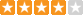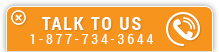Mass Balance Methodology

Course Number: M-2078
Credit: 2 PDH
Subject Matter Expert: Mark H. Otterson, P.E.
Price: \$59.90 Purchase using Reward Tokens. Details8 reviews
Overview

In Mass Balance Methodology, you'll learn ...

• A methodology for solving mass balance problems in steady-state continuous process systems
• How to solve mass balance problems with multiple streams
• How to solve mass balance problems with multiple process steps
• How chemical reactions are handled in mass balancing

OverviewPreview a portion of this course before purchasing it.

Credit: 2 PDH

Length: 28 pages

This course provides a methodology for solving mass balance problems in steady-state* continuous process systems. Mass balances are the important first step in essentially all chemical engineering problems for determining the flow rates, compositions and other key characteristics for each process stream. This information serves as the basis for essentially all subsequent process design calculations.

*A steady-state continuous process is one where the flows and compositions of each stream remain constant. Every atom that enters the defined process envelope must exit. There is no accumulation or consumption of matter within the closed process system.

The course begins with two elementary examples:

• Simple blending of two process streams to form one stream
• Simple separation of one process stream into two streams

Additional complexity is then introduced in a stepwise fashion:

• Multiple streams
• Multiple process steps
• Bypass streams

Finally, chemical reactions are considered:

• Mass balances are required for each atom
• Excess reactants & incomplete reactions

The following methodology is used in each mass balance problem:

• A process flow diagram (PFD) is drawn with each stream identified and given a unique label. Process "envelopes" are defined and indicated on the process flow diagram.
• A table is constructed. All of the known characterisitcs for each stream are included in the table.
• Mass balance equations are developed for the boundaries of each process envelope. This often results in a system of linear equations.
• The system of equations is solved using algebraic and/or matrix methods.

At the completion of this course, the student will be able to set up and solve relatively complex mass balance problems for steady-state continuous process systems.

Specific Knowledge or Skill Obtained

This course teaches the following specific knowledge and skills:

• How to set up and solve relatively complex mass balance problems for steady-state continuous process systems
• Simple blending processes
• Multiple feed streams
• Mass balancing a simple separation process
• Mass balancing methodology involving combustion of fuel

Certificate of Completion

You will be able to immediately print a certificate of completion after passing a multiple-choice quiz consisting of 15 questions. PDH credits are not awarded until the course is completed and quiz is passed.

Board Acceptance
 This course is applicable to professional engineers in: Alabama (P.E.) Alaska (P.E.) Arkansas (P.E.) Delaware (P.E.) Florida (P.E. Area of Practice) Georgia (P.E.) Idaho (P.E.) Illinois (P.E.) Illinois (S.E.) Indiana (P.E.) Iowa (P.E.) Kansas (P.E.) Kentucky (P.E.) Louisiana (P.E.) Maine (P.E.) Maryland (P.E.) Michigan (P.E.) Minnesota (P.E.) Mississippi (P.E.) Missouri (P.E.) Montana (P.E.) Nebraska (P.E.) Nevada (P.E.) New Hampshire (P.E.) New Jersey (P.E.) New Mexico (P.E.) New York (P.E.) North Carolina (P.E.) North Dakota (P.E.) Ohio (P.E. Self-Paced) Oklahoma (P.E.) Oregon (P.E.) Pennsylvania (P.E.) South Carolina (P.E.) South Dakota (P.E.) Tennessee (P.E.) Texas (P.E.) Utah (P.E.) Vermont (P.E.) Virginia (P.E.) West Virginia (P.E.) Wisconsin (P.E.) Wyoming (P.E.)
Reviews (8)
More DetailsPreview a portion of this course before purchasing it.

Credit: 2 PDH

Length: 28 pages